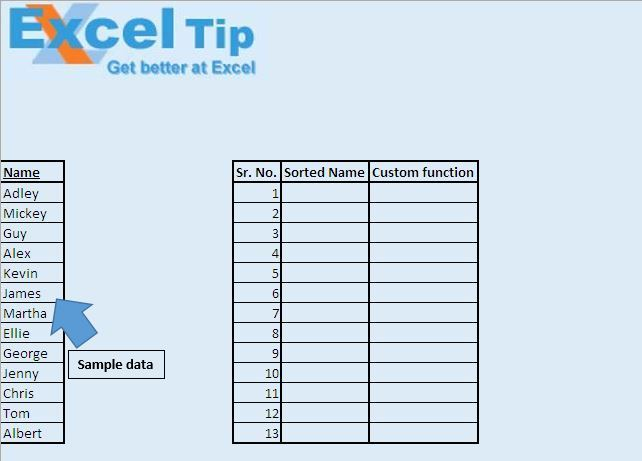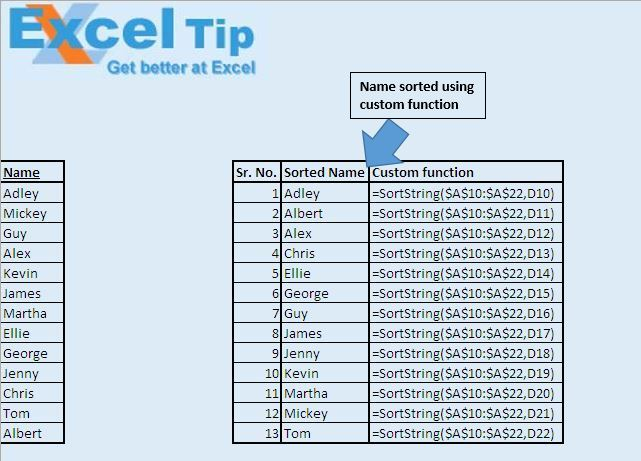# Sorting text data in alphabetical order using VBA in Microsoft Excel

In this article, we will create a custom function to sort data in alphabetical order.

Raw data consists of random names that we want to sort.Logic explanation

We have created “SortString” custom function to sort data alphabetically. It takes source range and position as inputs, and returns values based on the specified position. This function firstly creates a sorted array and then the specified position is used to extract the required value from the sorted array.Code explanation

UBound(values)

The above function is used to get the count of number of values within the array.

values(j) = values(j – 1)

The above code is used to shift values within the array by one index.

```
Option Explicit

Function SortString(Source As Range, Position As Long) As String

'Declaring variables
Dim Cell As Range
Dim values() As String
Dim i As Long, j As Long
Dim Done As Boolean

ReDim values(1 To 1)

'Looping through all cells in the range
For Each Cell In Source
Done = False
i = 1

'Creating an array of sorted name
Do
If Cell.Value < values(i) Then
Done = True
Else
i = i + 1
End If
Loop While Done = False And i <= UBound(values)

ReDim Preserve values(1 To UBound(values) + 1)

If i <= UBound(values) Then
'Adjusting value in sorted name array
For j = UBound(values) To i + 1 Step -1
values(j) = values(j - 1)
Next j
End If
values(i) = Cell.Value
Next Cell

'Assigning value of required position
SortString = values(Position + 1)

End Function

```